# Ordered semi-group

A semi-group equipped with a (generally speaking, partial) order $\le$ which is stable relative to the semi-group operation, i.e. for any elements $a,b,c$ it follows from $a \le b$ that $ac \le bc$ and $ca \le cb$. If the relation $\le$ on the ordered semi-group $S$ is a total order, then $S$ is called a totally ordered semi-group (cf. also Totally ordered set). If the relation $\le$ on $S$ defines a lattice (with the associated operations join $\vee$ and meet $\wedge$) satisfying the identities $$c(a \vee b) = ca \vee cb\ \ \text{and}\ \ (a \vee b)c = ac \vee bc$$ then $S$ is called a lattice-ordered semi-group; thus, the class of all lattice-ordered semi-groups, considered as algebras with semi-group and lattice operations, is a variety (cf. also Variety of groups). On a lattice-ordered semi-group the identities $$c(a \wedge b) = ca \wedge cb\ \ \text{and}\ \ (a \wedge b)c = ac \wedge bc$$ generally speaking, are not required to hold, and their imposition singles out a proper subvariety of the variety of all lattice-ordered semi-groups.

Ordered semi-groups arise by considering different numerical semi-groups, semi-groups of functions and binary relations, semi-groups of subsets (or subsystems of different algebraic systems, for example ideals in rings and semi-groups), etc. Every ordered semi-group is isomorphic to a certain semi-group of binary relations, considered as an ordered semi-group, where the order is set-theoretic inclusion. The classical example of a lattice-ordered semi-group is the semi-group of all binary relations on an arbitrary set.

In the general theory of ordered semi-groups one can distinguish two main developments: the theory of totally ordered semi-groups and the theory of lattice-ordered semi-groups. Although every totally ordered semi-group is lattice-ordered, both theories have developed to a large degree independently. The study of totally ordered semi-groups is devoted to properties that are to a large extent not shared by lattice-ordered semi-groups, while in considering lattice-ordered semi-groups one studies as a rule properties which, when applied to totally ordered semi-groups, reduce to degenerate cases. An important type of semi-groups is formed by the ordered groups (cf. Ordered group); their theory forms an independent part of algebra. In distinction to ordered groups, the order relation on an arbitrary ordered semi-group $S$ is, generally speaking, not defined by the set of its positive elements (i.e. the elements $a$ such that $ax \ge x$ and $xa \ge x$ for any $x$).

## Totally ordered semi-groups.

A semi-group $S$ is called orderable if one can define on it a total order which turns it into a totally ordered semi-group. A necessary condition for orderability is the absence in the semi-group of non-idempotent elements of finite order. If in an orderable semi-group the set of all idempotents is non-empty, then it is a sub-semi-group. Among the orderable semi-groups are the free semi-groups, the free commutative semi-groups, and the free $n$-step nilpotent semi-groups. There exists a continuum of methods for ordering free semi-groups of finite rank $\ge 2$. Certain necessary and sufficient conditions for the orderability of arbitrary semi-groups have been found, as well as for semi-groups from a series of known classes (e.g. semi-groups of idempotents, inverse semi-groups).

The structure of totally ordered semi-groups of idempotents has been completely described; in particular, the decomposition of such semi-groups into semi-lattices of rectangular semi-groups (cf. Idempotents, semi-group of) whose rectangular components are singular while the corresponding semi-lattices are trees. The completely-simple totally ordered semi-groups are exhausted by the right groups and the left groups and are lexicographic products of totally ordered groups and totally ordered semi-groups of right (respectively, left) zeros. By applying the reduction to totally ordered groups, a description of totally ordered semi-groups has been obtained in terms of the class of Clifford semi-groups, as well as a characterization in this way of the inverse totally ordered semi-groups (cf. Inversion semi-group). All types of totally ordered semi-groups generated by two mutually inverse elements have been classified (cf. Regular element).

The conditions imposed in the study of totally ordered semi-groups often postulate additional connections between the operation and the order relation. In this way one distinguishes the following basic types of totally ordered semi-groups: Archimedean semi-groups, naturally totally ordered semi-groups (cf. Naturally ordered groupoid), positive ordered semi-groups (in which all elements are positive), integral totally ordered semi-groups (in whichfor all). Every Archimedean naturally totally ordered semi-group is commutative; their structure is completely described. The structure of an arbitrary totally ordered semi-group is to a large extent determined by the peculiarities of its decomposition into Archimedean classes (cf. Archimedean class). For a periodic totally ordered semi-group this decomposition coincides with the decomposition into torsion classes, and, moreover, each Archimedean class is a nil semi-group. An arbitrary totally ordered nil semi-group is the union of an increasing sequence of convex nilpotent sub-semi-groups; in particular, it is locally nilpotent.

A homomorphism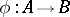of totally ordered semi-groups is called an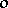-homomorphism ifis an isotone mapping fromto. A congruence (cf. Congruence (in algebra)) on a totally ordered semi-group is called an-congruence if all its classes are convex subsets; the kernel congruences of-homomorphisms are precisely the-congruences. The decomposition of a totally ordered semi-groupinto Archimedean classes does not always define-congruences, i.e. they are not always a band (cf. Band of semi-groups), but this is so, for example, ifis periodic and its idempotents commute or ifis a positive totally ordered semi-group.

For a totally ordered semi-group there arises an additional condition of simplicity (cf. Simple semi-group), related to the order. One such condition is the lack of proper convex ideals (convex ideally-simple, or-simple, semi-groups); a trivial example of such a totally ordered semi-group is a totally ordered group. A totally ordered semi-groupwith a least elementand a greatest element(in particular, finite) will be convex ideally simple if and only ifandare at the same time left and right zeros in. Any totally ordered semi-group may be imbedded, while preserving the order (-isomorphically), in a convex ideally-simple totally ordered semi-group. There exist totally ordered semi-groups with cancellation, non-imbeddable in a group, but a commutative totally ordered semi-group with cancellation can be-isomorphically imbedded in an Abelian totally ordered group; moreover, there exists a unique group of fractions, up to an-isomorphism. A totally ordered semi-group is-isomorphically imbeddable in the additive group of real numbers if and only if it satisfies the cancellation law and contains no abnormal pair (i.e. elements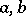such that either,for all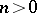, or,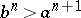for all).

## Lattice-ordered semi-groups.

If for two elementsandin an ordered semi-group there exists a greatest elementwith the property, then it is called a right quotient and is denoted by, the left quotient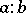is defined similarly. A lattice-ordered semi-groupis called a lattice-ordered semi-group with division if the right and left quotients exist infor any pair of elements. Such semi-groups are complete (as a lattice) lattice-ordered semi-groups, their lattice zero is also the multiplicative zero and they satisfy the infinite distributive laws,. An important example of a lattice-ordered semi-group with division is the multiplicative semi-group of ideals of an associative ring, and a notable direction in the theory of lattice-ordered semi-groups deals with the transfer of many properties and results from the theory of ideals in associative rings to the case of lattice-ordered semi-groups (the unique decomposition into prime factors, primes, primary, maximal, principal elements of a lattice-ordered semi-group, etc.). For example, the well-known relation of Artin in the theory of commutative rings can be translated as follows in the theory of lattice-ordered semi-groups with division and having a one 1: Let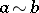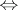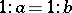. If the lattice-ordered semi-groupbeing considered is commutative, then the relationis a congruence on it; moreover, the quotient semi-group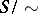is a (lattice-ordered) group if and only ifis integrally closed, i.e.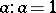for every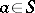.

The study of lattice-ordered semi-groups is connected with groups by considering the imbedding problems of a lattice-ordered semi-group in a lattice-ordered group. For example, every lattice-ordered semi-group with cancellation and the Ore condition (cf. Imbedding of semi-groups) and whose multiplication is distributive relative to both lattice operations, is imbeddable in a lattice-ordered group.

The theory of lattice-ordered semi-groups has begun to be studied from the point of view of the theory of varieties: the free lattice-ordered semi-groups have been described, the minimal varieties of lattice-ordered semi-groups have been found, etc.